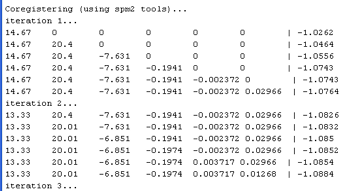# DTI Data Handling

Jump to: navigation, search

This section includes several useful functions for handling DTI data.

#  General Format of DTI Data

DTI data in our code is usually stored as a multidimensional array in one of the following two formats:

• Image format XxYxZxpxN: Coordinates are, respectively:
1. X image dimension
2. Y image dimension
3. Z image dimension
4. multivariate dimension (e.g. p=6 for the 6 tensor entries, or p=3 for the 3 principal diffusion direction entries)
5. subjects
• Indexed format nxpxN: Coordinates are, respectively:
1. single image dimension (see dtiImgToInd.m, below)
2. multivariate dimension (e.g. p=6 for the 6 tensor entries, p=3 for the 3 principal diffusion direction entries, or p=1 for an FA map.)
3. subjects

#  Volume Compression

DTI datasets are large. Substantial compression can be achieved by simply extracting those voxels that belong to the mask of interest.

• dtiImgToInd.m: Converts a DTI array in image XxYxZxpxN format to indexed nxpxN format by extracting the n voxels in the mask.
• dtiIndToImg.m: Converts a DTI array in indexed nxpxN format to image XxYxZxpxN format by reassigning the n voxels according to the mask.

Both functions also work with a DTI structure with fields .dt6 (tensor array in dt6 format), .val (array of eigenvalues), .vec (array of eigenvectors), and .fa (array of FA values).

#  Basic Computations

• dti33to6.m: Converts DTI data in symmetric 3-by-3 matrix format to dt6 format, i.e. the 6-element vector [D11 D22 D33 D12 D13 D23].
• dt6to33.m: Converts DTI data in the 6-element vector format dt6 to the symmetric 3-by-3 matrix format.
• dtiEig.m: Eigenvalue decomposition, computes eigenvalues and eigenvectors of each tensor in the array.
• dtiEigComp.m: Eigenvalue composition, recomputes tensor from eigenvalues and eigenvectors at each element of the array.
• dtiComputeFA.m: Computes FA from array of eigenvalues.

#  Transforming ROIs from mrVista

Often, you will define an ROI in the course of fMRI analyses and want to use the ROI in your DTI analyses. In order to 'see' such functionally-defined ROIs in dtiFiberUI, the ROIs need to be transformed.

1. Load the ROI of interest in mrVista
2. Open the Volume 3-view window (Inplane 1: Window -> Open volume 3-view window).
3. In the new volume window, transform the ROI (Volume 1: Xform -> Inplane to volume -> ROIs). It will now appear in the ROI menu of the volume window.
4. Save the ROI (Volume 1: File -> ROI -> Save ROI). It will be stored in subject/Volume/ROIs
5. Start dtiFiberUI, and compute a mrVista transform (dtiFiberUI: Xform -> Compute mrVista xform). This process requests a vAnatomy file and requires the spm2 toolbox. It will take a few minutes and spit numbers to the commmand window like this:6. Import the ROI from mrVista (dtiFiberUI: Xform -> import mrVista volume ROI)
7. Save the ROI in desired DTI/ROIs directory
• Note: try not to close any of the mrVista, volume, or dtiFiberUI windows until you are done transforming between them